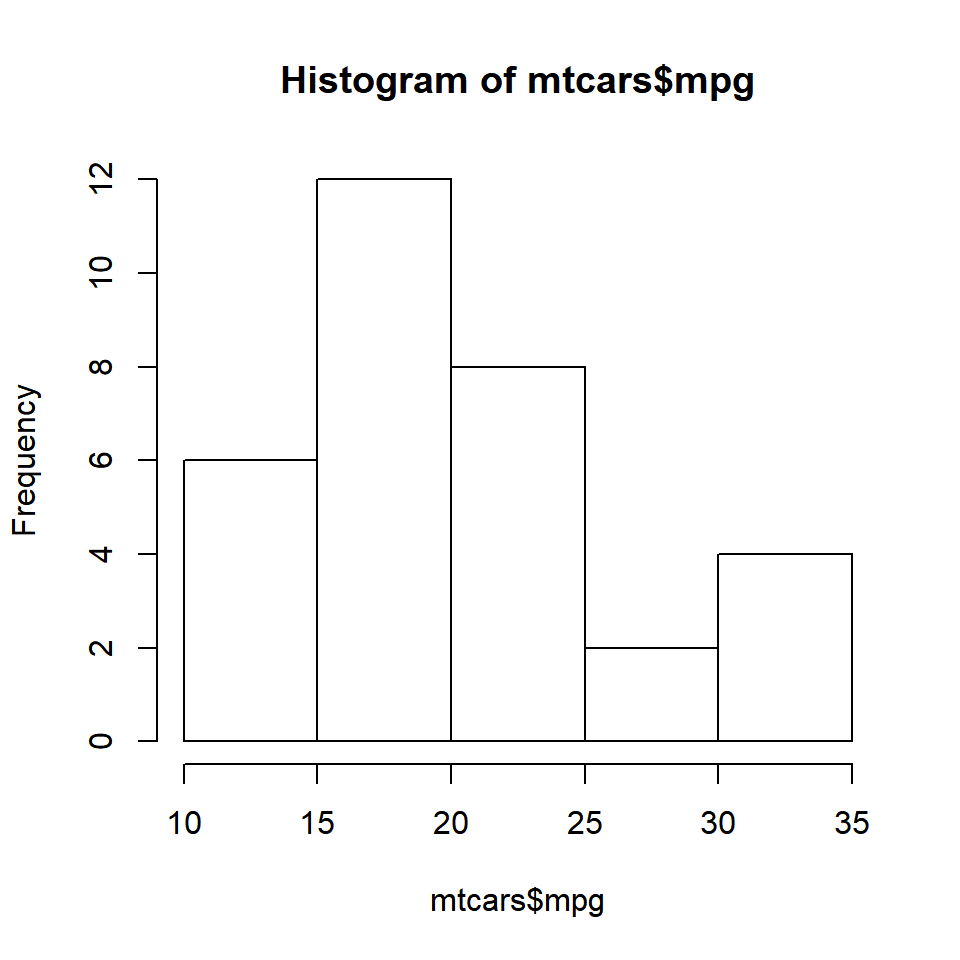## Agenda

Learn to construct and use histograms to examine the underlying distribution of a continuous variable. Specifically

• create a bare bones histogram
• specify the number of bins/intervals
• represent frequency density on the Y axis
• add colors to the bars and the border
• add labels to the bars

## Introduction

A histogram is a plot that can be used to examine the shape and spread of continuous data. It looks very similar to a bar graph and can be used to detect outliers and skewness in data. The histogram graphically shows the following:

• center (location) of the data
• spread (dispersion) of the data
• skewness
• outliers
• presence of multiple modes

## Histograms

To construct a histogram

• the data is split into intervals called bins
• the intervals may or may not be equal sized
• for each bin, the number of data points that fall into it are counted (frequency)
• the Y axis of the histogram represents the frequency and
• the X axis represents the variable

## Histogram## Histogram

``h <- hist(mtcars\$mpg)``